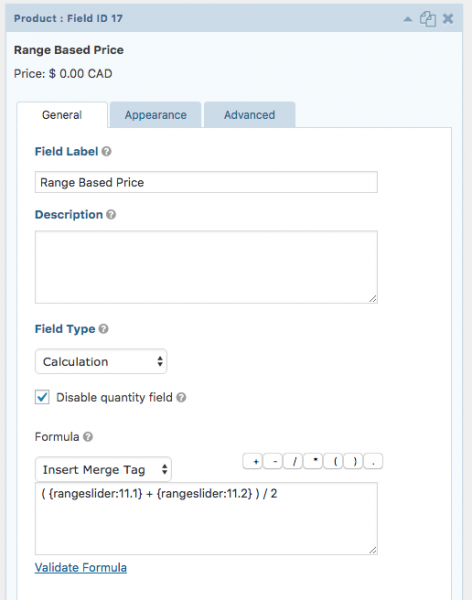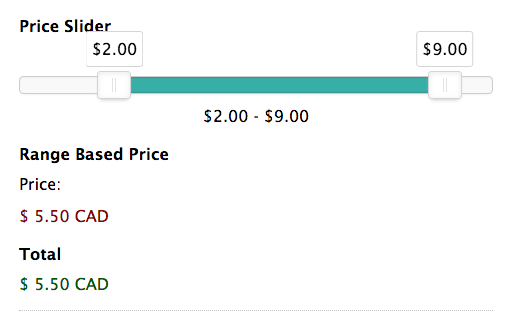# Using with Pricing Fields

You can use the Gravity Forms Range Slider in conjunction with a Product field to affect the price of your product.

In this example, we’ll take the median value of the two slider handles to create a final price.

First, under Formatting, we set the Prefix to include the “\$” sign, then set the Decimals to “2”.Next, we add a Product field below our slider and set its Field Type to “Calculation”.

In the Formula area, our Range Slider “min” and “max” fields should be visible in the “Insert Merge Tags” dropdown (click “Update” if you do not see these fields ).

For this example, we added the merge tag for the “min” value, then added the it with the “max” value, then divided them by 2.The final formula would be:

`( {rangeslider:11.1} + {rangeslider:11.2} ) / 2`

This will now use the sliders values to calculate the total of your form.#### What Else Can I Do with the GF Range Slider?